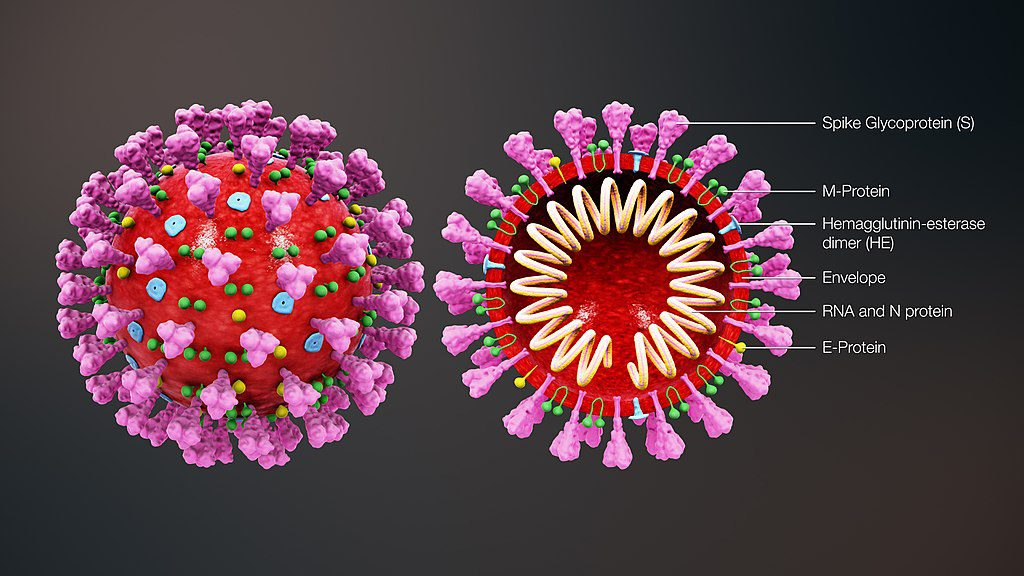# How to generate a Coronavirus 3D geometric model?

How to generate a Coronavirus 3D geometric model like this one:Or this one:## Attempt

Here is some related code:

Block[{ang = -0.9, dia = 0.04, ext = 2.4, turn = 20, r = 0.42},
spring = ParametricPlot3D[
r*{(ext + Cos[(2 turn x)/(1 - ang)]) Cos[x],
Sin[(2 turn x)/(
1 - ang)], (ext + Cos[(2 turn x)/(1 - ang)]) Sin[x]}, {x,
0, (1 - ang) \[Pi]},
PlotStyle -> {Lighter[Yellow], Tube[dia]}][[1, 1]];
]

shell = RegionPlot3D[
2 <= x^2 + y^2 + z^2 <= 3 && (y > -0.5), {x, -2, 2}, {y, -2,
2}, {z, -2, 2}, PlotStyle -> Red, Mesh -> None,
PlotPoints -> 100, PlotTheme -> "Minimal"][[1, 1]];

Graphics3D[{shell, spring}, Boxed -> False, Background -> Black]For the spiral formulas I used the code from this demonstration by Sandor Kabai: "Helical Spring between Two Cylinders".

• @Shredderroy Yes, to get Coronavirus 3D graphs I started by looking into "spiky" posts in MSE. (Like this one.) – Anton Antonov Apr 3 '20 at 15:37
• This could be of some help: 119324 – exp ikr Apr 5 '20 at 15:04
• @expikx Thanks, that is a great suggestion! – Anton Antonov Apr 6 '20 at 1:11
• – Jason B. Apr 12 '20 at 20:02
• also you could try Import["https://www.dropbox.com/sh/uj2n1yjj301gl9j/AAAPg2L-\ EDrE3mcuMzRlSEiWa/Covid%2019.stl?dl=1", "STL"] but the resulting region is too large for the FE to do anything with. – Jason B. Apr 12 '20 at 20:05

The problem of arranging four kinds of elements without intersection on a sphere has many solutions. I will indicate one of them. First, we will depict the entire sphere assuming that the elements are equally and their total number is 300:

p = SpherePoints;
p2 = Table[p[[2 i]], {i, 150}];
p1 = Table[p[[2 i - 1]], {i, 150}];
pS = Table[p2[[2 i]], {i, 75}]; pM = Table[p1[[2 i]], {i, 75}]; pE =
Table[p1[[2 i - 1]], {i, 75}];
pHE = Table[p2[[2 i - 1]], {i, 75}];
r1 = Sqrt; r0 = Sqrt; r2 =
r0 + 2.7 (Sqrt - Sqrt); dr = 0.04;

cylS = Table[{Pink, Cylinder[{r0 pS[[i]], r2 pS[[i]]}, dr]}, {i,
Length[pS]}];
sphS = {Pink, Sphere[r2 pS, 2 dr]};
cylE = Table[{Yellow, Cylinder[{r0 pE[[i]], r1 pE[[i]]}, dr/2]}, {i,
Length[pE]}];
sphE = {Yellow, Sphere[(r1 + 2 dr) pE, 2 dr]}; sphM = {Green,
Sphere[(r1 + 2 dr) pM, 2 dr]}; sphM1 =
Rotate[sphM, 5 dr/r1, {1, 1, 1}]; cylHE =
Table[{LightBlue, Cylinder[{r0 pHE[[i]], r1 pHE[[i]]}, dr]}, {i,
Length[pHE]}];
cylHEt = Table[{LightGreen,
Cylinder[{r1 pHE[[i]], (r1 + dr) pHE[[i]]}, 2 dr]}, {i,
Length[pHE]}];

Graphics3D[{{Red, Sphere[{0, 0, 0}, r1]}, cylS, sphS, cylE, sphE,
cylHE, cylHEt, sphM, sphM1}, Boxed -> False, Background -> Black,
Lighting -> "Neutral"]Cross section with RNA and penetrating elements:

Block[{ang = -0.9, dia = 0.04, ext =2.4, turn = 20, r = 0.42},
spring = ParametricPlot3D[
r*{(ext + Cos[(2 turn x)/(1 - ang)]) Cos[x],
Sin[(2 turn x)/(1 - ang)], (ext +
Cos[(2 turn x)/(1 - ang)]) Sin[x]}, {x, 0, (1 - ang) \[Pi]},
PlotStyle -> {Lighter[Yellow], Tube[dia]}][[1, 1]];]

shell = RegionPlot3D[
r0^2 <= x^2 + y^2 + z^2 <= r1^2 && (y > -0.5), {x, -2, 2}, {y, -2,
2}, {z, -2, 2}, PlotStyle -> Red, Mesh -> None,
PlotPoints -> 100, PlotTheme -> "Minimal"][[1, 1]];

{Graphics3D[{shell, spring, cylS, sphS, cylE, sphE, cylHE, cylHEt,
sphM, sphM1}, Boxed -> False, Background -> Black,
PlotRange -> {All, {-.0, 2}, All}],
Graphics3D[{shell, spring, cylS, sphS, cylE, sphE, cylHE, cylHEt,
sphM, sphM1}, Boxed -> False, Background -> Black,
PlotRange -> {All, {-.5, 2}, All}]}• Excellent, thank you! – Anton Antonov Apr 14 '20 at 1:19
• @AntonAntonov You are welcome! – Alex Trounev Apr 14 '20 at 10:15
• @AntonAntonov I am sorry, but spring is apparently your code. I just used it without changing. Also check code for broken expressions (now it is a problem of this forum). I fixed the code. Try again. – Alex Trounev Apr 14 '20 at 21:09
• Just accepted this answer — I should have done that some time ago... – Anton Antonov Sep 26 '20 at 21:19
• @AntonAntonov Thank you! Лучше поздно, чем никогда:) – Alex Trounev Sep 26 '20 at 21:34

## UPDATE

I recommend taking a look at the following post:

3D Modeling of the SARS-CoV-2 Virus in the Wolfram Language https://community.wolfram.com/groups/-/m/t/1989540

## ORIGINALOne can also try to import 3D parts created by other people. If you search online you can probably find some sites. For instance, from here

https://3dprint.nih.gov/discover/coronavirus

you can get a model and combine it with your spiral (a bit resized):

obj=Import["https://wolfr.am/LNm1PWwN"];

spring=Block[{ang=-0.9,dia=1,ext=2.4,turn=20,r=10},
ParametricPlot3D[r{
(ext+Cos[(2 turn x)/(1-ang)]) Cos[x],
Sin[(2 turn x)/(1-ang)]+.5,
(ext+Cos[(2 turn x)/(1-ang)]) Sin[x]},
{x,0,(1-ang) \[Pi]},PlotStyle->{Lighter[Yellow],Tube[dia]}]];

Show[{obj,spring},PlotRange->{{-50,50},{-10,50},{-50,50}},ImageSize->400{1,1}]
`
• Thank you for your answer and the 3dprint link! – Anton Antonov Apr 14 '20 at 13:18